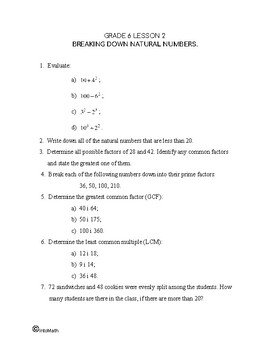CA$2.00 In this lesson you will learn and practice a very important skill of dividing decimals using long division. For example, dividing 2.34 by 2 or 34.5 by 0.4. It is important to understand the place value well and how it affects the final answer. Sometimes it makes sense to convert a decimal into a fraction to perform division. 0.2 is the same as 2/10 84 divided by 0.2 is the same as 84 multiplied by 10/2, which results in 420.Grade 6 Workbook (16 pages with answers) CA$4.00

You will also learn how decimals are related to unit conversions (between systems and within each system – metric, imperial, etc).

We focus on converting  metric units of length and mass – cm to m, kg to g and so on. For example, 1000 grams is equal to 1 kilogram, thus 0.001 kilogram is the same as 1 gram.

Metric system became popular in Europe around 1800-s. Merchants required a better system to conduct trade. The old system was replaced by a decimal system, based on kilogram and meter.